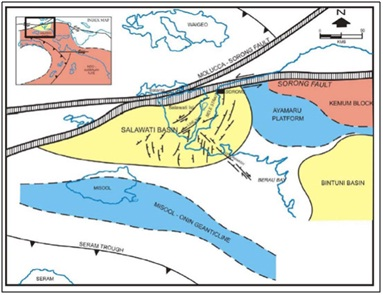# Rock Physics Formula and RMS Stacking Velocity Calculation to Assist Acoustic Impedance Inversion that Constrain Well Data

• Handoyo Institut Teknologi Sumatera
• Mochammad Puput Erlangga Geophysical Engineering, Institut Teknologi Bandung, Indonesia
• Paul Young TGS NOPEC Geophysical Company ASA, Perth
Keywords: Acoustic impedance, rock physics, stacking velocity, wellog

### Abstract

This research ilustrate the generation of acoustic impedance inversion in the absence of well log using stacking velocity input in Salawati Basin, Papua, Indonesia using data obtained from seismic lines and stacking velocity section. Initial acoustic impedance modelswere first before the inversion process and were created by spreading the value of well log data to the all seismic CDP. The calculated acoustic impedance logs from standard sonic and density logs were used to build the initial model of acoustic impedance.First, the stacking velocities was first interpolated on a grid that has the same size as the seismic data using by means of Polynomial algorithm. This was closely followed by the conversion of the stacking velocities to interval velocities using Dix’s equation. The matrix densities were estimated by simple rock physics approach i.e. Gardner’s equation as a velocity function. The initial model of acoustic impedance was calculated by multiplying the densities section and interval velocities section. The resulting initial model of acoustic impedance was inverted to obtain the best of acoustic impedance section based on reflectivity.

### References

Lindseth, R. O., 1979, Synthetic sonic logs – a process for stratigraphic interpretation: Geophysics, Vol. 44, No. 1.

Lloyd, H. J. E., and Margrave, G. F., 2012, Well tying and trace balancing Hussar data using new MATLAB tools: CREWES Research Report, Vol 24.

Margrave, G. F., 2002, Methods of Seismic Data Processing Lecture Notes Geophysics 577: University of Calgary.

Mavko G., Mukerji T. and Dvorkin J. 2009. The Rock Physics Handbook: Tools for Seismic Analysis in Porous Media. Cambridge University Press.

Oldenburg, D. W., Levy, S. and Stinson, K., Root-mean-square velocities and recovery of the acoustic impedance. Geophysics, Vol. 49 No. 10. 1653-1663.

Satyana, A. H., 2003, Sorong Fault and Reversal of the Salawati Basin: Indonesian Petroleum Association Newsletter, Jakarta, March 2003.

Situmeang, M., 2012, Karakteristik Reservoar Karbonat Menggunakan Inversi Sparse Spike di Lapangan ”Panda” Formasi Kais Cekungan Salawati, Papua [unpublished]: Yogyakarta, Universitas Pembangunan Nasional “Veteran”, 76p.Published
2020-06-24
Section
Research Articles
Abstract viewed = 19 times